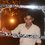# Help Please..

1) A polynomial $$p(x)={ x }^{ 4 }+a{ x }^{ 3 }+b{ x }^{ 2 }+cx+d$$ has roots $$\sqrt { 2 } ,e$$ and $$\pi$$ and no other roots. Let $$I=\int _{ \sqrt { 2 } }^{ e }{ p(x)dx }$$ and $$J=\int _{ e }^{ \pi }{ p(x)dx. }$$ Then,

(A) $I$ and $J$ must have opposite signs.

(B) $I$ and $J$ can be both positive but not both negative.

(C) $I$ and $J$ can be both negative but not both positive.

(D) We do not have enough information to compare the signs of $I$ and $J$.

2) All the inner angles of a -7 gon are obtuse , their sizes in degree being distinct integers divisible by 9. What is the sum(in degree) of the largest two angles?

(A) 300

(B)315

(C)330

(D)335Note by Anandhu Raj
5 years, 7 months ago

This discussion board is a place to discuss our Daily Challenges and the math and science related to those challenges. Explanations are more than just a solution — they should explain the steps and thinking strategies that you used to obtain the solution. Comments should further the discussion of math and science.

When posting on Brilliant:

• Use the emojis to react to an explanation, whether you're congratulating a job well done , or just really confused .
• Ask specific questions about the challenge or the steps in somebody's explanation. Well-posed questions can add a lot to the discussion, but posting "I don't understand!" doesn't help anyone.
• Try to contribute something new to the discussion, whether it is an extension, generalization or other idea related to the challenge.
• Stay on topic — we're all here to learn more about math and science, not to hear about your favorite get-rich-quick scheme or current world events.

MarkdownAppears as
*italics* or _italics_ italics
**bold** or __bold__ bold
- bulleted- list
• bulleted
• list
1. numbered2. list
1. numbered
2. list
Note: you must add a full line of space before and after lists for them to show up correctly
paragraph 1paragraph 2

paragraph 1

paragraph 2

[example link](https://brilliant.org)example link
> This is a quote
This is a quote
    # I indented these lines
# 4 spaces, and now they show
# up as a code block.

print "hello world"
# I indented these lines
# 4 spaces, and now they show
# up as a code block.

print "hello world"
MathAppears as
Remember to wrap math in $$ ... $$ or $ ... $ to ensure proper formatting.
2 \times 3 $2 \times 3$
2^{34} $2^{34}$
a_{i-1} $a_{i-1}$
\frac{2}{3} $\frac{2}{3}$
\sqrt{2} $\sqrt{2}$
\sum_{i=1}^3 $\sum_{i=1}^3$
\sin \theta $\sin \theta$
\boxed{123} $\boxed{123}$

## Comments

Sort by:

Top Newest

1. C and 2. B

- 5 years, 7 months ago

Log in to reply

Could you please explain how it come through?

- 5 years, 7 months ago

Log in to reply

For the first ques as it has three roots and no other root and also the function is bi quadratic so there must be a repeated root. Considering three different cases just plot the graph and check the sign of integral.

- 5 years, 7 months ago

Log in to reply

1.C we can verify it by graph

2.B because the angles would be 99,108,117,126,135,153,162 as all angles are obtuse and divisible by 9 so sum of 2 largest angles =153+162=315

Btw U too preparing for kvpy??

- 5 years, 7 months ago

Log in to reply

Yup!! By the way could you please help with these also? The positive integer k for which $\frac { { 101 }^{ \frac { k }{ 2 } } }{ k! }$ is a maximum is?

- 5 years, 7 months ago

Log in to reply

×

Problem Loading...

Note Loading...

Set Loading...